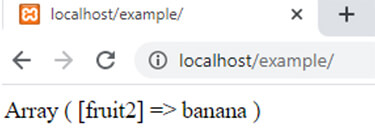array_diff_ukey() function in php || PHP Functions# array_diff_ukey() function in php

1552

array_diff_ukey() function of PHP is used to calculate the difference of two or more arrays by comparing the keys of that arrays with the help of a user built function.

Syntax:

array_diff_ukey(\$arr1,\$arr2,....,\$arrN,function);

Here

1. \$arr1 is the array which different values return by the function.

2. \$arr2 and \$arrN are the arrays which keys are compared with the first array.

3. function is the user define function which compare the keys of arrays and calculate the result.

Example:

```<?php
function user_function(\$x,\$y)
{
if (\$x===\$y)
{
return 0;
}
return (\$x>\$y)?1:-1;
}
\$arr1=array("fruit1"=>"mango","fruit2"=>"banana","fruit3"=>"pear");
\$arr2=array("fruit4"=>"orange","fruit1"=>"mango","fruit3"=>"grapes");
\$result=array_diff_ukey(\$arr1,\$arr2,"user_function");
print_r(\$result);
?>```

Output:Share:## Big Hosting Days

Upto 75@ OFF on Web Hosting

## Get a .COM for just \$6.98

Secure Domain for a Mini Price